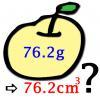#### You may also like### Far Horizon

An observer is on top of a lighthouse. How far from the foot of the lighthouse is the horizon that the observer can see?### Data Matching

Use your skill and judgement to match the sets of random data.### More or Less?

Are these estimates of physical quantities accurate?

# Big and Small Numbers in the Physical World

##### Age 14 to 16Challenge Level

Think about how best to approximate these things from the physical world around us. You will need to make some estimations and find information from friends or other sources, as would any scientist! Take care to represent all of your answers using a sensible number of decimal places and be sure to note all of your assumptions clearly.

1. Light travels at $c=3\times 10^8$ metres per second. How fast in this in miles per hour? How many times faster is this than a sports car?
2. The Milky Way is a spiral galaxy with diamater about 100,000 light years and thickness about 1000 light years. There are estimated to be between 100 billion and 400 billion stars in the galaxy. Estimate the average distance between these stars.
3. Density of lead $11.34$g/cm$^3$. How big would a tonne of lead be?
4. Estimate the mass of ore it takes to produce a roll of aluminum kitchen foil.
5. How many AA batteries contain enough charge between them to run a laptop for an hour?
6. Estimate how many atoms there are in a staple.
7. Einstein's equation tells us that the enegy $E$ stored in matter equals $mc^2$, where $m$ is the mass and $c$ is the speed of light. How much energy is contained in the staple from question 6? How long could this energy run your laptop for?
8. How much energy would it take to raise the air temperature of the room you are in by 1$^\circ$C? How much gas must be burned to produce this much energy? What is the cost of that much gas?

NOTES AND BACKGROUND
An obvious part of the skill with applying mathematics to physics is to know the fundamental formulae and constants relevent to a problem. By not providing these pieces of information directly, you need to engage at a deeper level with the problems. You might not necessarily know all of the required formulae, but working out which parts you can and cannot do is all part of the problem solving process!